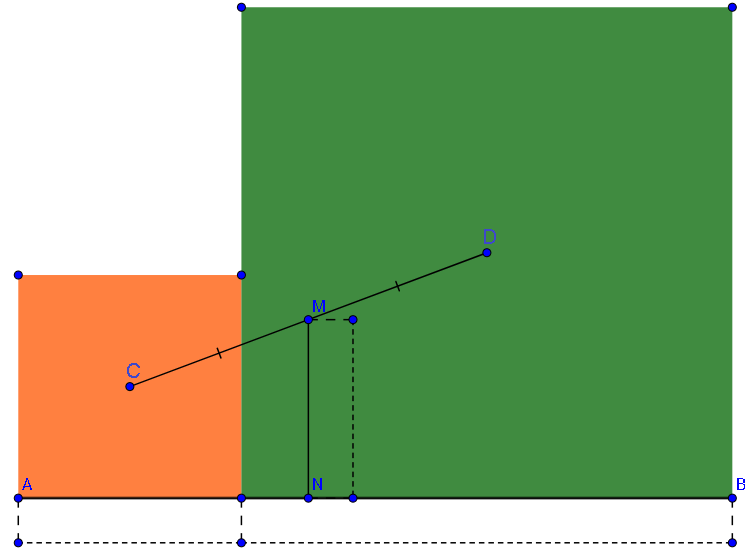# Squares!

Geometry Level 2$C$ and $D$ are the centers of the orange square and the green square. $M$ is the midpoint of $CD$. $MN \perp AB$. Calculate $\frac{AB}{MN}$

This is part of the series: "It's easy, believe me!"

×

Problem Loading...

Note Loading...

Set Loading...